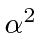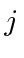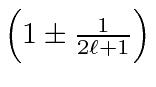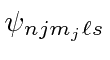## The Fine Structure of Hydrogen

We have solved the problem of a non-relativistic, spinless electron in a coulomb potential exactly. Real Hydrogen atoms have several small corrections to this simple solution. If we say that electron spin is a relativistic effect, they can all be called relativistic corrections which are off ordercompared to the Hydrogen energies we have calculated.

1. The relativistic correction to the electron's kinetic energy.
2. The Spin-Orbit correction.
3. The Darwin Term'' correction to s states from Dirac equation.

Calculating these fine structure effects separately and summing them we find that we get a nice cancellation yielding a simple formula.The correction depends only on the total angular quantum number and does not depend onso the states of different total angular momentum split in energy but there is still a good deal of degeneracy. It makes sense, for a problem with spherical symmetry, that the states of definite total angular momentum are the energy eigenstates and that the result depend on.

We also compute the Zeeman effect in which an external magnetic field is applied to Hydrogen. The external field is very important since it breaks the spherical symmetry and splits degenerate states allowing us to understand Hydrogen through spectroscopy.

The correction due to a weak magnetic field is found to beThe factoris known as the LandeFactorbecause the state splits as if it had this gyromagnetic ratio. We know that it is in fact a combination of the orbital and spin g factors in a state of definite. We have assumed that the effect of the field is small compared to the fine structure corrections. We can write the full energy in a weak magnetic field.Thus, in a weak field, the the degeneracy is completely broken for the states. All the states can be detected spectroscopically.

In the strong field limit we could use states of definiteandand calculate the effects of the fine structure,, as a perturbation. In an intermediate strength field, on the order of 500 Gauss, the combination of the Hydrogen fine structure Hamiltonian and the term to the B field must be diagonalized on the set of states with the same principal quantum number.

Jim Branson 2013-04-22